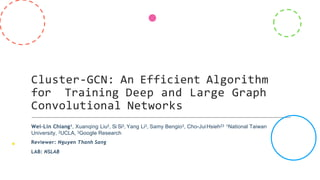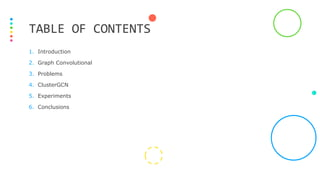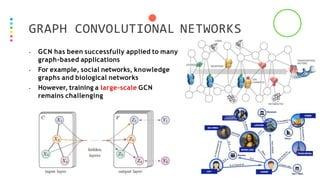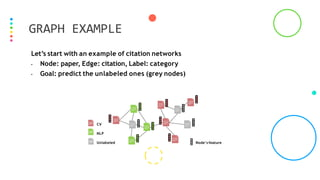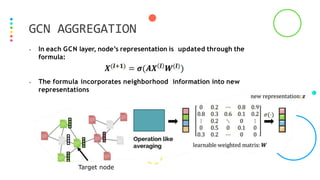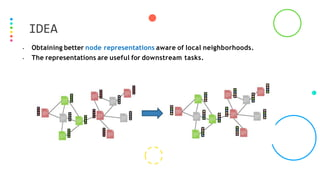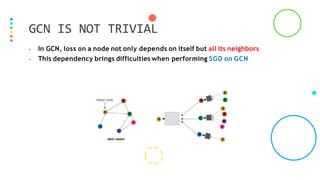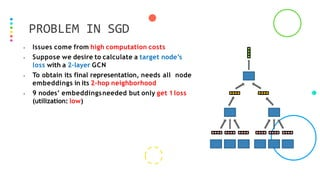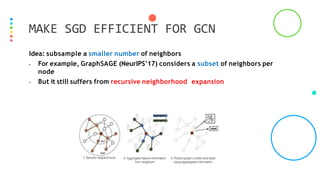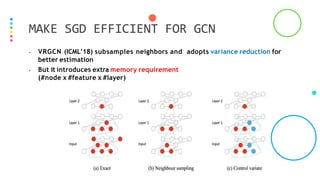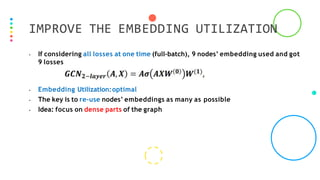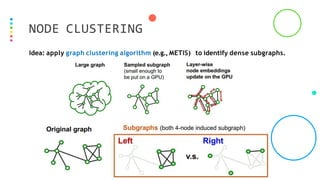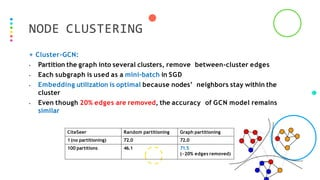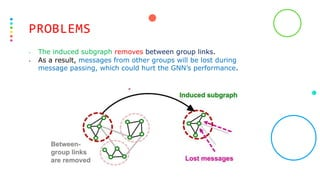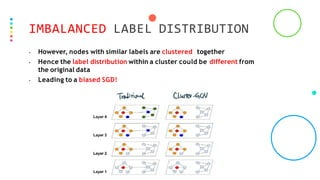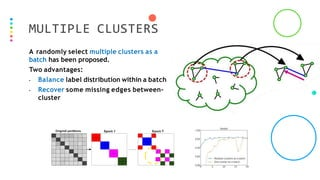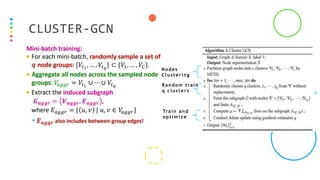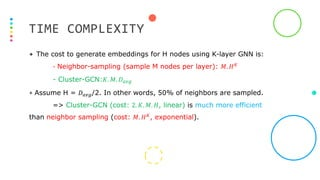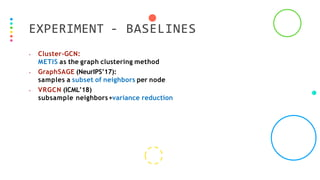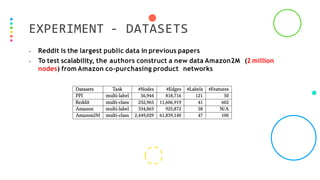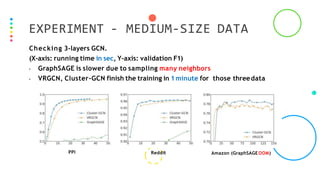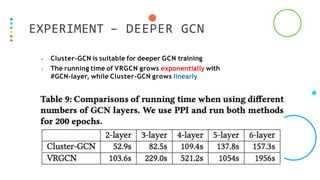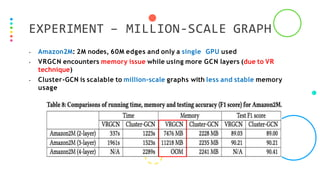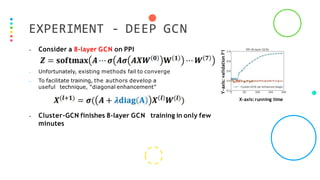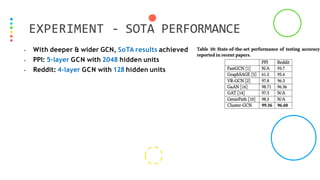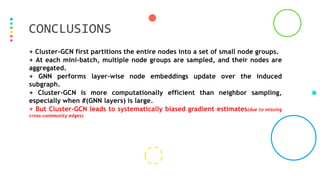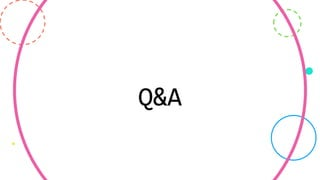1 of 28

### NS-CUK Joint Journal Club: S.T.Nguyen, Review on “Cluster-GCN: An Efficient Algorithm for Training Deep and Large Graph Convolutional Networks”, KDD 2019

1. Cluster-GCN: An Efficient Algorithm for Training Deep and Large Graph Convolutional Networks Wei-Lin Chiang1, Xuanqing Liu2, Si Si3, Yang Li3, Samy Bengio3, Cho-JuiHsieh23 1National Taiwan University, 2UCLA, 3Google Research Reviewer: Nguyen Thanh Sang LAB: NSLAB
2. 1. Introduction 2. Graph Convolutional 3. Problems 4. ClusterGCN 5. Experiments 6. Conclusions TABLE OF CONTENTS
3. GRAPH CONVOLUTIONAL NETWORKS • GCN has been successfully applied to many graph-based applications • For example, social networks, knowledge graphs and biological networks • However, training a large-scale GCN remains challenging
4. GRAPH EXAMPLE Let’s start with an example of citation networks • Node: paper, Edge: citation, Label: category • Goal: predict the unlabeled ones (grey nodes) CV NLP Node’s feature Unlabeled
5. GCN AGGREGATION • In each GCN layer, node’s representation is updated through the formula: • The formula incorporates neighborhood information into new representations Target node
6. IDEA • Obtaining better node representations aware of local neighborhoods. • The representations are useful for downstream tasks.
7. GCN IS NOT TRIVIAL • In GCN, loss on a node not only depends on itself but all its neighbors • This dependency brings difficulties when performing SGD on GCN
8. PROBLEM IN SGD • Issues come from high computation costs • Suppose we desire to calculate a target node’s loss with a 2-layer GCN • To obtain its final representation, needs all node embeddings in its 2-hop neighborhood • 9 nodes’ embeddingsneeded but only get 1loss (utilization: low)
9. MAKE SGD EFFICIENT FOR GCN Idea: subsample a smaller number of neighbors • For example, GraphSAGE (NeurIPS’17) considers a subset of neighbors per node • But it still suffers from recursive neighborhood expansion
10. MAKE SGD EFFICIENT FOR GCN • VRGCN (ICML’18) subsamples neighbors and adopts variance reduction for better estimation • But it introduces extra memory requirement (#node x #feature x #layer)
11. IMPROVE THE EMBEDDING UTILIZATION • If considering all losses at one time (full-batch), 9 nodes’ embedding used and got 9 losses • Embedding Utilization:optimal • The key is to re-use nodes’ embeddings as many as possible • Idea: focus on dense parts of the graph
12. NODE CLUSTERING Idea: apply graph clustering algorithm (e.g., METIS) to identify dense subgraphs.
13. NODE CLUSTERING + Cluster-GCN: • Partition the graph into several clusters, remove between-cluster edges • Each subgraph is used as a mini-batch in SGD • Embedding utilization is optimal because nodes’ neighbors stay within the cluster • Even though 20% edges are removed, the accuracy of GCN model remains similar CiteSeer Random partitioning Graph partitioning 1(no partitioning) 72.0 72.0 100 partitions 46.1 71.5 (~20% edges removed)
14. PROBLEMS • The induced subgraph removes between group links. • As a result, messages from other groups will be lost during message passing, which could hurt the GNN’s performance.
15. IMBALANCED LABEL DISTRIBUTION • However, nodes with similar labels are clustered together • Hence the label distribution within a cluster could be different from the original data • Leading to a biased SGD!
16. MULTIPLE CLUSTERS A randomly select multiple clusters as a batch has been proposed. Two advantages: • Balance label distribution within a batch • Recover some missing edges between- cluster
17. CLUSTER-GCN Nodes Clustering Random train q clusters Train and optimize
18. TIME COMPLEXITY + The cost to generate embeddings for H nodes using K-layer GNN is: - Neighbor-sampling (sample M nodes per layer): 𝑀. 𝐻𝐾 - Cluster-GCN:𝐾. 𝑀. 𝐷𝑎𝑣𝑔 + Assume H = 𝐷𝑎𝑣𝑔/2. In other words, 50% of neighbors are sampled. => Cluster-GCN (cost: 2. 𝐾. 𝑀. 𝐻, linear) is much more efficient than neighbor sampling (cost: 𝑀. 𝐻𝐾, exponential).
19. EXPERIMENT - BASELINES • Cluster-GCN: METIS as the graph clustering method • GraphSAGE (NeurIPS’17): samples a subset of neighbors per node • VRGCN (ICML’18) subsample neighbors+variance reduction
20. EXPERIMENT - DATASETS • Reddit is the largest public data in previous papers • To test scalability, the authors construct a new data Amazon2M (2 million nodes) from Amazon co-purchasing product networks
21. EXPERIMENT - MEDIUM-SIZE DATA Checking 3-layers GCN. (X-axis: running time in sec, Y-axis: validation F1) • GraphSAGE is slower due to sampling many neighbors • VRGCN, Cluster-GCN finish the training in 1minute for those threedata PPI Reddit Amazon (GraphSAGEOOM)
22. EXPERIMENT – DEEPER GCN • Cluster-GCN is suitable for deeper GCN training • The running time of VRGCN grows exponentially with #GCN-layer, while Cluster-GCN grows linearly
23. EXPERIMENT – MILLION-SCALE GRAPH • Amazon2M: 2M nodes, 60M edges and only a single GPU used • VRGCN encounters memory issue while using more GCN layers (due to VR technique) • Cluster-GCN is scalable to million-scale graphs with less and stable memory usage
24. EXPERIMENT - DEEP GCN X-axis:running time Y-axis: validation F1 • Consider a 8-layer GCN on PPI • Unfortunately, existing methods fail to converge • To facilitate training, the authors develop a useful technique, “diagonal enhancement” • Cluster-GCN finishes 8-layer GCN training in only few minutes
25. EXPERIMENT - SOTA PERFORMANCE • With deeper & wider GCN, SoTA results achieved • PPI: 5-layer GCN with 2048 hidden units • Reddit: 4-layer GCN with 128 hidden units
26. CONCLUSIONS + Cluster-GCN first partitions the entire nodes into a set of small node groups. + At each mini-batch, multiple node groups are sampled, and their nodes are aggregated. + GNN performs layer-wise node embeddings update over the induced subgraph. + Cluster-GCN is more computationally efficient than neighbor sampling, especially when #(GNN layers) is large. + But Cluster-GCN leads to systematically biased gradient estimates(due to missing cross-community edges)
27. Q&A
28. THANK YOU!